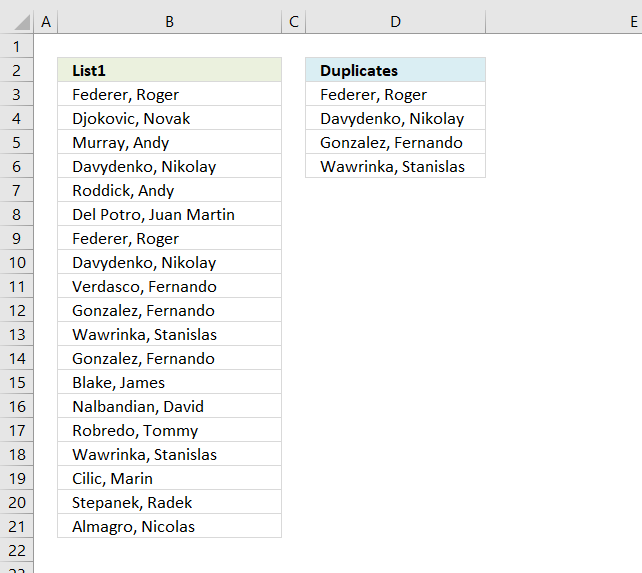Author: Oscar Cronquist Article last updated on February 28, 2019

The array formula in cell C2 extracts duplicate values from column A. Only one duplicate of each value is displayed in column C.Update 2017-08-19! New regular formula in cell D3:

=LOOKUP(2,1/(((COUNTIF(\$F\$2:F2, \$B\$3:\$B\$21)=0)*(COUNTIF(\$B\$3:\$B\$21, \$B\$3:\$B\$21)>1))), \$B\$3:\$B\$21)

#### This video explains how to use the formula and how it works

The following formula is an outdated formula, the above formula is smaller and better.

Array formula in D3:

=INDEX(\$B\$3:\$B\$21, MATCH(0, COUNTIF(D2:\$D\$2, \$B\$3:\$B\$21)+IF(COUNTIF(\$B\$3:\$B\$21, \$B\$3:\$B\$21)>1, 0, 1), 0))

#### How to enter an array formula

1. Copy (Ctrl + c) above formula
2. Double press with left mouse button on cell C2
3. Paste (Ctrl + v) to cell C2
4. Press and hold CTRL + SHIFT simultaneously
5. Press Enter once
6. Release all keys

Your formula now looks like this: {=array_formula}
Don't enter the curly brackets, they appear automatically.

A beginners guide to Excel array formulas

Array formulas allows you to do advanced calculations not possible with regular formulas.

#### How to copy the formula

Copy cell C2 and paste it to cells below as far as needed.

#### Remove #num errors:

=IFERROR(INDEX(\$B\$3:\$B\$21, MATCH(0, COUNTIF(D2:\$D\$2, \$B\$3:\$B\$21)+IF(COUNTIF(\$B\$3:\$B\$21, \$B\$3:\$B\$21)>1, 0, 1), 0)), "")

Copy cell C2 and paste it down to D20.

How to use the IFERROR function

The IFERROR function lets you catch most errors in Excel formulas. It was introduced in Excel 2007. In previous Excel […]

#### Earlier Excel versions, array formula in C2:

=IF(ISERROR(INDEX(\$A\$2:\$A\$20, MATCH(0, COUNTIF(C1:\$C\$1, \$A\$2:\$A\$20)+IF(COUNTIF(\$A\$2:\$A\$20, \$A\$2:\$A\$20)>1, 0, 1), 0))), "", INDEX(\$A\$2:\$A\$20, MATCH(0, COUNTIF(C1:\$C\$1, \$A\$2:\$A\$20)+IF(COUNTIF(\$A\$2:\$A\$20, \$A\$2:\$A\$20)>1, 0, 1), 0)))

The IFERROR function was introduced in Excel 2007, if you have an earlier version then use the formula above.

### How (the old) array formula works

#### Step 1 - Show a duplicate value only once

=INDEX(\$B\$3:\$B\$21, MATCH(0, COUNTIF(D2:\$D\$2, \$B\$3:\$B\$21)+IF(COUNTIF(\$B\$3:\$B\$21, \$B\$3:\$B\$21)>1, 0, 1), 0))

COUNTIF(D2:\$D\$2, \$B\$3:\$B\$21) contains both a relative and absolute reference (D2:\$D\$2) to a range.

When you copy a cell reference like this the cell reference expands.

COUNTIF(D2:\$D\$2, \$B\$3:\$B\$21) returns this array in cell C2: (0, 0, 0, 0, 0, 0, 0, 0, 0, 0, 0, 0, 0, 0, 0, 0, 0, 0, 0, 0)

How to use the COUNTIF function

Counts the number of cells that meet a specific condition.

#### Step 2 - Filter values in \$A\$2:\$A\$20 having duplicates

=INDEX(\$B\$3:\$B\$21, MATCH(0, COUNTIF(D2:\$D\$2, \$B\$3:\$B\$21)+IF(COUNTIF(\$B\$3:\$B\$21, \$B\$3:\$B\$21)>1, 0, 1), 0))

COUNTIF(\$B\$3:\$B\$21, \$B\$3:\$B\$21) counts the number of cells within a range that meet the given condition. The returning array is (2, 1, 1, 2, 1, 1, 2, 2, 1, 2, 2, 1, 2, 1, 1, 2, 1, 1, 1, 1).

COUNTIF(\$B\$3:\$B\$21, \$B\$3:\$B\$21)>1 returns this array: (TRUE, FALSE, FALSE, TRUE, FALSE, FALSE, TRUE, TRUE, FALSE, TRUE, TRUE, FALSE, TRUE, FALSE, FALSE, TRUE, FALSE, FALSE, FALSE, FALSE).

IF(COUNTIF(\$B\$3:\$B\$21, \$B\$3:\$B\$21)>1, 0, 1) returns (1, 0, 0, 1, 0, 0, 1, 1, 0, 1, 1, 0, 1, 0, 0, 1, 0, 0, 0, 0).

How to use the IF function

Checks if a logical expression is met. Returns a specific value if TRUE and another specific value if FALSE.

#### Step 3 - Calculate arrays combined

=INDEX(\$B\$3:\$B\$21, MATCH(0, COUNTIF(D2:\$D\$2, \$B\$3:\$B\$21)+IF(COUNTIF(\$B\$3:\$B\$21, \$B\$3:\$B\$21)>1, 0, 1), 0))

COUNTIF(D2:\$D\$2, \$B\$3:\$B\$21)+IF(COUNTIF(\$B\$3:\$B\$21, \$B\$3:\$B\$21)>1, 0, 1)

(0, 0, 0, 0, 0, 0, 0, 0, 0, 0, 0, 0, 0, 0, 0, 0, 0, 0, 0, 0) + (1, 0, 0, 1, 0, 0, 1, 1, 0, 1, 1, 0, 1, 0, 0, 1, 0, 0, 0, 0) equals

(1, 0, 0, 1, 0, 0, 1, 1, 0, 1, 1, 0, 1, 0, 0, 1, 0, 0, 0, 0)

#### Step 4 - Identify duplicates

=INDEX(\$B\$3:\$B\$21, MATCH(0, COUNTIF(D2:\$D\$2, \$B\$3:\$B\$21)+IF(COUNTIF(\$B\$3:\$B\$21, \$B\$3:\$B\$21)>1, 0, 1), 0))

MATCH(0, COUNTIF(D2:\$D\$2, \$B\$3:\$B\$21)+IF(COUNTIF(\$B\$3:\$B\$21, \$B\$3:\$B\$21)>1, 0, 1), 0) becomes

MATCH(0, (1, 0, 0, 1, 0, 0, 1, 1, 0, 1, 1, 0, 1, 0, 0, 1, 0, 0, 0, 0), 0) is 2.

Match returns the relative position of an item in an array that matches a specified value

How to use the MATCH function

Identify the position of a value in an array.

#### Step 5 - Return duplicates

=INDEX(\$B\$3:\$B\$21, MATCH(0, COUNTIF(D2:\$D\$2, \$B\$3:\$B\$21)+IF(COUNTIF(\$B\$3:\$B\$21, \$B\$3:\$B\$21)>1, 0, 1), 0))

becomes

=INDEX(\$B\$3:\$B\$21, 2) is Federer, Roger.

Index returns a value or reference of the cell at the intersection of a particular row and column, in a given range

How to use the INDEX function

Gets a value in a specific cell range based on a row and column number.

#### Final thoughts

When formula in c2 is copied to c3 the reference changes.

Example

The formula in c2: =INDEX(\$A\$2:\$A\$20, MATCH(0, COUNTIF(D2:\$D\$2, \$A\$2:\$A\$20)+IF(COUNTIF(\$A\$2:\$A\$20, \$A\$2:\$A\$20)>1, 0, 1), 0))

Then copy the formula to C3.

The formula references changes: =INDEX(\$A\$2:\$A\$20, MATCH(0, COUNTIF(D3:\$D\$2, \$A\$2:\$A\$20)+IF(COUNTIF(\$A\$2:\$A\$20, \$A\$2:\$A\$20)>1, 0, 1), 0))